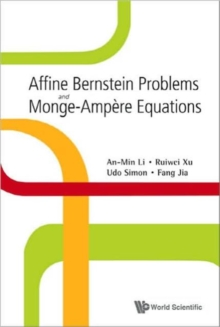Supporting your high street Find out how »
• My AccountAffine Bernstein Problems And Monge-ampere Equations Hardback

Description

In this monograph, the interplay between geometry and partial differential equations (PDEs) is of particular interest.

It gives a selfcontained introduction to research in the last decade concerning global problems in the theory of submanifolds, leading to some types of Monge-Ampere equations.From the methodical point of view, it introduces the solution of certain Monge-Ampere equations via geometric modeling techniques.

Here geometric modeling means the appropriate choice of a normalization and its induced geometry on a hypersurface defined by a local strongly convex global graph.

For a better understanding of the modeling techniques, the authors give a selfcontained summary of relative hypersurface theory, they derive important PDEs (e.g. affine spheres, affine maximal surfaces, and the affine constant mean curvature equation).

Concerning modeling techniques, emphasis is on carefully structured proofs and exemplary comparisons between different modelings.

Information

• Format: Hardback
• Pages: 192 pages
• Publisher: World Scientific Publishing Co Pte Ltd
• Publication Date:
• Category: Differential calculus & equations
• ISBN: 9789812814166

£71.00

£58.55

on all orders

Pick up orders

from local bookshops